Equations
Exponents
Order of Operations
Percent
Misc.
100

5x=-105

-21

100

24

16

100

Simplify the following expression: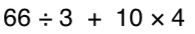100

-4 + -5

9100

What is 3/4 as a percent?

75%

100

What are the factors of 20?

1, 2, 4, 5, 10, 20

200

p-54 =36

90

200

What is 9^3 (exponent)

729

200

Simplify the following expression: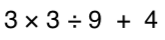200

14 + -12

2

https://www.khanacademy.org/math/arithmetic/arith-review-negative-numbers/arith-review-add-negatives-intro/v/adding-negative-numbers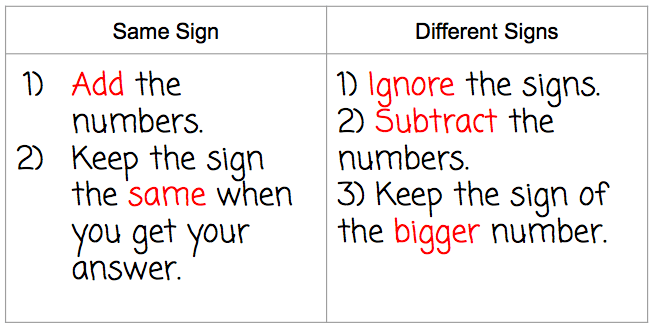200

50% of 298 is what number?

149

200

What are the factors of 68?

1, 2, 4, 17, 34, 68

300

3x+1=-8

x=-3

300

what is 2^5

32

300

3 + 42 * (3 + 2)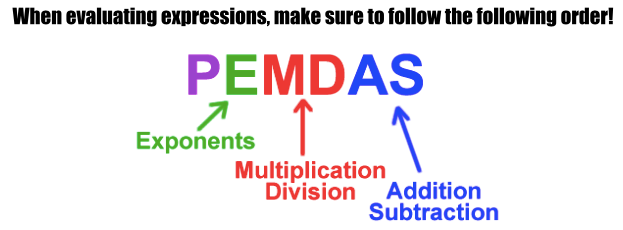300

-10 + 15

5300

95% is what as a fraction in simplest form?

19/20

300

What is the greatest common factor of 64, 16, 32

16

400

6+ k/4 = 14

32

400

What is the value of:

84

4,096

400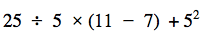400

-18 + (-12) =

-30

400

What percent of 224 is 56?

25%

400

Find the Least common Multiple of 14, 21 and 7

42

500

27 =5x +2

x=5

500500

6 + (-2 + -5)

-1

500

12.5 is what percent of 1250?

1%

500

Find the Least Common Multiple of 3, 12, 6

12

Click to zoom